## Comparison of Different Learning Types in Machine Learning

### Lists of subfields:

• Transfer learning (迁移学习)

• Multi-task learning (多任务学习)

• Meta learning (元学习)

• Curriculum learning (课程学习)

• Online learning (在线学习)

• (optional) On-the-job learning

### 1. Transfer Learning

[A very helpful introduction: here]

Transfer learning is about how to use a pre-trained network and apply it to custom task, transferring what it learned from previous tasks.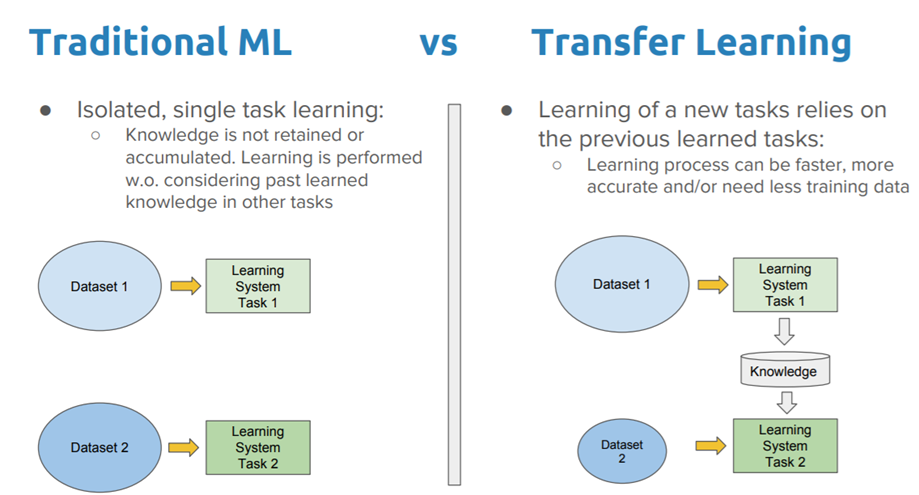### 2. Multi-task Learning

[A very helpful introduction: here]

Multi-task learning refers to multiple learning tasks are solved at the same time, while exploiting commonalities and differences across tasks. As soon as you find yourself optimizing more than one loss function, you are effectively doing multi-task learning (in contrast to single-task learning).

Two most commonly used ways to perform multi-task learning are hard and soft parameter sharing of hidden layers. Hard parameter sharing greatly reduces the risk of overfitting.

#### 2.1 Hard parameter sharing

Hard parameter sharing is the most commonly used approach. It is generally applied by sharing the hidden layers between all tasks, while keeping several task-specific output layers.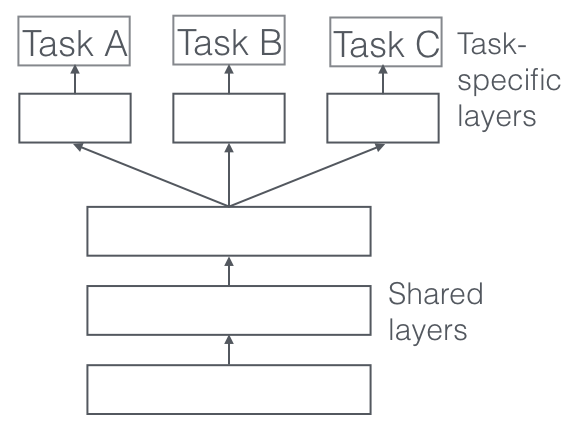#### 2.2 Soft parameter sharing

In soft parameter sharing, each task has its own model with its own parameters. The distance between the parameters of the model is then regularized in order to encourage the parameters to be similar.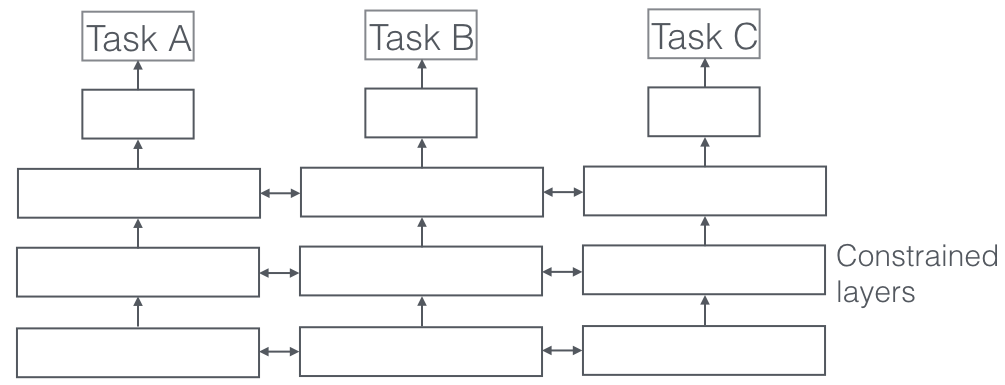### 3. Meta Learning

[A very helpful introduction: here]

Meta learning also means learning to learn. It is inspired by the fact that humans have an intrinsic ability to learn new skills quickly. A typical scenerio where meta learning be applied is few-shot learning.

Here is an example. Let’s say we want to solve a few-shot classification problem. In training data, there are 5 fruit classes and each class has only 1 example. We are supposed to classify new images as belonging to one of these classes. A conventional supervised learning model would take training data as input and make prediction for test data, during which parameters of the model are updated through backpropagation.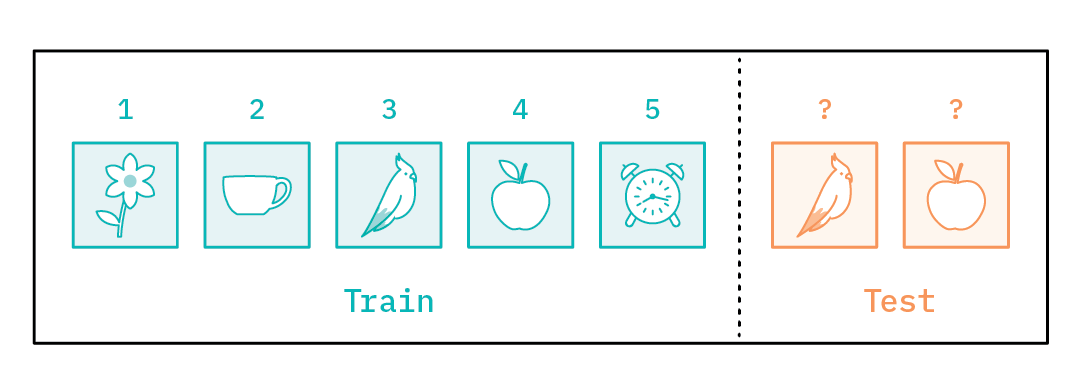But one problem is that with only five images available for training, the model would likely overfit and perform poorly on the test images. One way to solve the problem is using a pre-trained network from other tasks, and then fine-tune it on the five training images. This is how meta learning works.

Meta learning process looks like this. Each training example that is comprised of pairs of train and test data points is called an episode. This is different from the way that data is set up for conventional supervised learning. The training data (also called the meta-training data) is composed of train and test examples, alternately referred to as the support and query set. Meta-learning also has meta-validation and meta-test sets.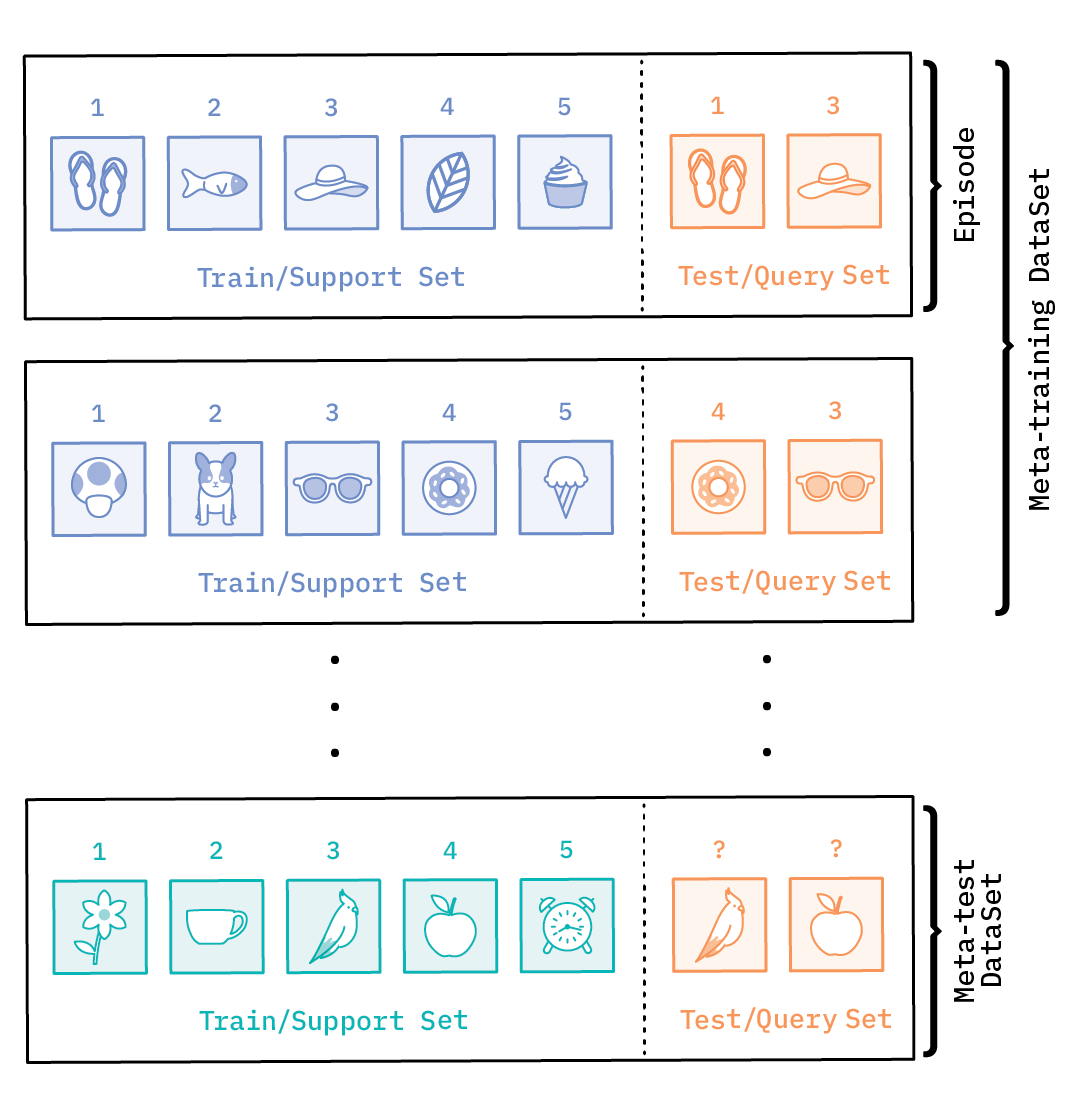How meta-training process works is like, firstly learning a model based on eposide1, then testing on query set of eposide2 using its support set. Recurse this process on different eposides. So that's how the model is learnt. Later we can apply this model on meta-validation data and meta-test data for testing.

Difference between transfer learning and meta learning: Transfer learning tries to optimize configurations for a model and meta learning simply reuses an already optimized model, or part of it at least.

### 4. Curriculum Learning

[A very helpful introduction: here]

Curriculum learning describes a type of learning in which you first start out with only easy examples of a task and then gradually increase the task difficulty. The idea of training neural networks with a curriculum was proposed by Jeffrey Elman in 1933. There are several categories of curriculum learning. Most cases are applied to Reinforcement Learning, with a few exceptions on Supervised Learning.

Here are five types of curriculum for reinforcement learning.### 5. Online Learning

Online machine learning is a method of machine learning in which data becomes available in a sequential order (streaming). And it is also known as incremental learning. Comparatively the convolutional learning is called offline learning.

Online learning aims to learn in real time. Thus, it's supposed to be significantly more efficient both in time and space with more practical batch algorithms, and the model is expected to change just as regularly to capture and adapt to new changes.